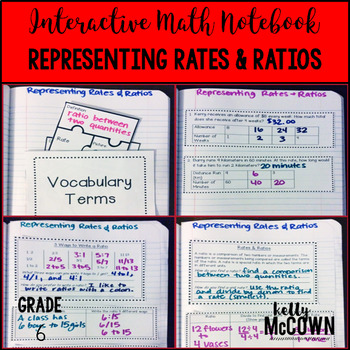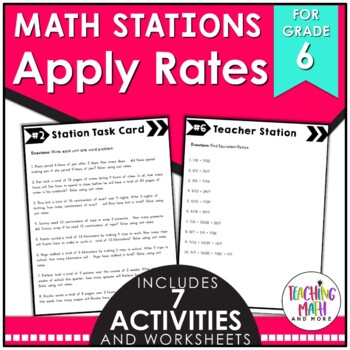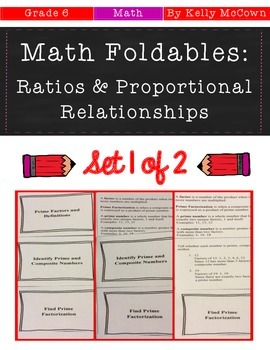## Friday, October 21, 2016

### Teaching Rates & Ratios {Grade 6}

One of the building skills that needs mastery for seventh grade is learning rates and ratios. In sixth grade students learn the foundations for how to solve proportions in seventh grade. Students have prior knowledge of numbers, expressions, and division to help them in learning how to solve rates and ratios.

I have listed here activities to review and practice Rates and Ratios. I hope these help jump start your lesson planning into the world of Rates and Ratios. The key to getting your students involved in the learning process is to include interactive, visual, engaging activities for them to actively participate in.These interactive notebook activities are intended to help students understand the concept of a ratio, use ratio language to describe a relationship between two quantities, understand the concept of a unit rate, use rate language to describe a ratio relationship, and use ratio and rate reasoning to solve real-world and mathematical problems.Included are:
-4 different Rates & Ratios activities to engage students
-Teacher facilitated activity for 60-90 minutes of classroom time
-Activities include: Vocabulary, Practice of Writing Ratio Tables, Reading Ratio Word Problems and Writing Ratios 3 Different Ways, and Finding Unit Rates
-Examples of Completed Interactive Math Notebook Activities are includedThese interactive notebook activities are intended to help students understand how to use ratio and rate reasoning to solve real-world and mathematical problems. Students will learn how to reason about tables of equivalent ratios, use double number line diagrams and equations..

Included are:
-4 different Rates & Ratios activities to engage students
-Teacher facilitated activity for 60-90 minutes of classroom time
-Activities include: Vocabulary, Practice of Writing Equivalent Ratios, Finding Unit Rates for different quantities, and Finding Unit Rate Prices of sales items.
-Examples of Completed Interactive Math Notebook Activities are includedThis math station activity is intended to help students understand the concept of a ratio, use ratio language to describe a relationship between two quantities, understand the concept of a unit rate, use rate language to describe a ratio relationship, and use ratio and rate reasoning to solve real-world and mathematical problems.Included are:
-6 different stations to engage students
-Teacher facilitated activity for 60-90 minutes of classroom time
-Stations include:
1. Vocabulary
3. Practice of Rates & Ratios
4. Additional Practice of Rates & Ratios
5. Real World applications of Ratios
6. Word problems of Rates & Ratios
-Student Station Guide helps students record their answersThis math station activity is intended to help students understand how to use ratio and rate reasoning to solve real-world and mathematical problems. Students will learn how to reason about tables of equivalent ratios, use double number line diagrams and equations.

Included are:
-6 different stations to engage students
-Teacher facilitated activity for 60-90 minutes of classroom time
-Stations include:
1. Vocabulary
3. Practice of Rates & Ratios
4. Additional Practice of Rates & Ratios
5. Real World applications of Ratios
6. Word problems of Rates & Ratios
-Student Station Guide helps students record their answersThese math foldables are for students to be able to understand the concept of a ratio, use ratio language, understand the concept of a unit rate, use ratio and rate reasoning to solve real-world and mathematical problems, make tables of equivalent ratios, and solve unit rate problems.

Included are:
-6 different NO PREP Math Foldables to engage students in note taking
-Teacher facilitated activity for 15-20 minutes of classroom time each
-PRINT & GO teach foldables that are ready with NO PREP
-Directions for using Math Foldables in your classroom
-Options for scaffolding with blank templates
-Foldables included:
Foldable: Ratios & Rates
Foldable: Ratio Tables
Foldable: Proportions
Foldable: Solving Proportions
Foldable: Sequence and Expressions
Foldable: Proportions and Equations
Foldable: Four Blank TemplatesThis November Math NO PREP packet that will keep your sixth graders engaged! This packet is just plain fun. Not only is it PACKED with sixth-grade common core math problems, it also gives students fun coloring, puzzles, and problem solving. Use this packet for bellwork, classwork, extra credit, fast finishers, or homework!

Topics Covered:
-Operations with Fractions
-Graphing on a Coordinate Plane
-Operations with Decimals
-Represent Ratios & Rates
-Apply Ratios & Rates
I hope these resources are helpful in getting your students engaged in Rates & Ratios. Keeping students actively engaged in the learning process will ensure that they are in charge of their own learning. The goal of learning Rates and Ratios is for students to develop a foundational knowledge to continue to grow upon in seventh grade.